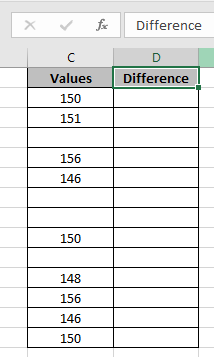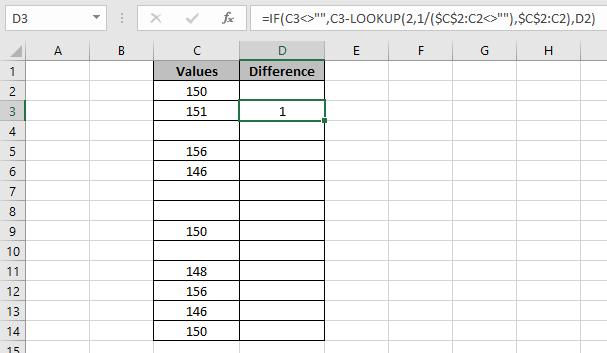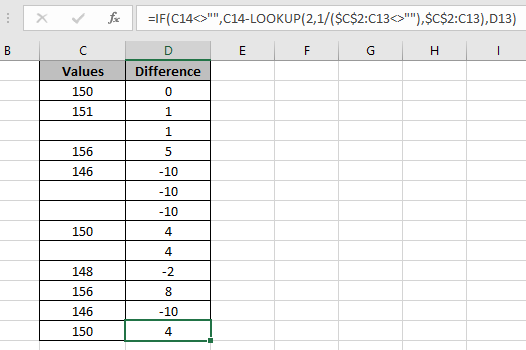# Difference with the last non-blank cell

In this article, we will learn how to make a difference with the last non-blank cell in Excel.

Let's first get some knowledge about the function first.
The LOOKUP function finds the estimated value in the range and returns the value from the range or its corresponding value.

Here, we will be using one of the properties of the LOOKUP function i.e. if LOOKUP function can't find an exact match, it returns the last & nearest value. It returns the last found value. We will be using the syntax below.

Syntax:

 = IF ( Cell <>"" , Cell - LOOKUP ( 2 , 1 / ( exp_range<>"" ), exp_range ) , last_result )

Here we will be using one more attribute of excel which is exp_ranges. Expanding ranges is a mixed reference where absolute reference (\$A\$1) and relative reference (A1) both are used.

Explanation:

1. Exp_range<>"" returns an array of TRUE and FALSE.
2. LOOKUP function considers TRUE as 1 and FALSE as 0.
3. 1 / ( exp_range<>"") when each value of array is under division with 1 returns an array of 1's and #VALUE! error.
4. Lookup value is 2, so the last found value of 1 in an array of 1's and #value error returns the last non-blank cell.
5. The function returns the difference if Cell <>"" condition stand TRUE or else it returns the last_result obtained.

Let’s understand it by an example.

Here we have some values measured and recorded results of it in a column. There are some blank cells among the data.Use the formula in the D4 cell:

 = IF ( C3<>"" , C3 - LOOKUP ( 2 , 1 / ( \$C\$2:C2<>"" ), \$C\$2:C2), D2)

Explanation:

• C3<>"" checks the cell is empty or not.
• \$C\$2:C2<>"" returns an array of TRUE and FALSE.
• LOOKUP function considers TRUE as 1 and FALSE as 0.
• 1 / ( \$C\$2:C2<>"" ) when each value of array is under division with 1 returns an array of 1's and #VALUE! Error.
• Lookup value is 2, So the last found value of 1 in an array of 1s and #VALUE! error returns the last non-blank cell.
• The function returns the difference if Cell <>"" condition stand TRUE or else it returns the last_result obtained.

Here, the range is given as expanding range and cell reference for the single cell reference.As you can see in the above snapshot the difference of 151 with 150 comes out to be 1. That seems fine. Now we will copy the formula to the rest of the cells using the Ctrl + D Shortcut or drag down cells option of excel.As you can see the formula in the last cell which is = IF ( C14<>"" , C14 - LOOKUP(2,1/(\$C\$2:C13<>"") , \$C\$2:C13) , D13 ) and you can see I added 0 at the start of the table to complete the table. Below are some of the observations about this formula.

Notes:

1. The formula returns #NA error if no match is found in the array.
2. The formula checks the lookup value first and then looks up for the just previous value, if the lookup value is not found.
3. All excel formula always returns the first value found in the table array.
4. The above-explained formula, lookup for the last file version in the filename array.

Hope you understood how to take Difference form the last non-blank cell in Excel. Explore more articles on LOOKUP functions here. Please feel free to state your queries below in the comment box. We will certainly help you.

Related Articles

How to use the IF function in Excel

How to use LOOKUP function in Excel

How to use the VLOOKUP function in Excel

How to use the HLOOKUP function in Excel

Popular Articles

Edit a dropdown list

If with conditional formatting

If with wildcards

Vlookup by date

1.This does not work reliably, as lookup only works on sorted data. It finds non-null, but sometimes gives wrong value. See the important note in the current Excel help for lookup inside excel (as of 23.02.2020)

•Hey Alex,

I agree with you on the reliability factor of LOOKUP function. I saw the important note in the current Excel help for lookup inside excel (as of 23.02.2020).
The array form of LOOKUP looks in the first row or column of an array for the specified value and returns a value from the same position in the last row or column of the array. The array form of LOOKUP is provided for compatibility with other spreadsheet programs, but it's functionality is limited. Recommend you to use the VLOOKUP function wherever required.

Terms and Conditions of use

The applications/code on this site are distributed as is and without warranties or liability. In no event shall the owner of the copyrights, or the authors of the applications/code be liable for any loss of profit, any problems or any damage resulting from the use or evaluation of the applications/code.